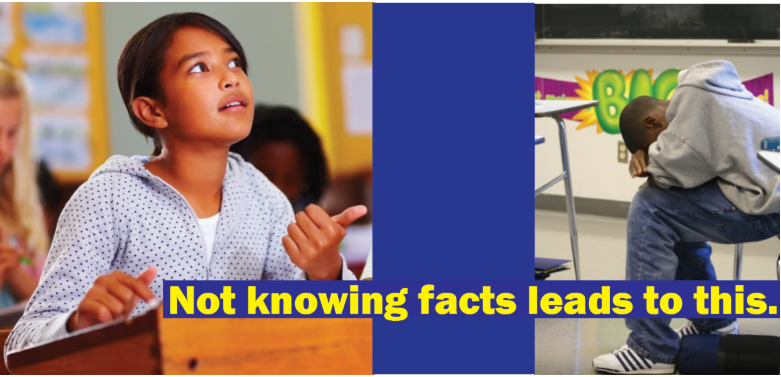## Why Teach Multiplication Facts to Struggling Pre-Algebra Students?

You want your middle-grade students to complete the pre-algebra math topics so they are ready to begin to study algebra in 8th or 9th grade. A disheartening number of middle-grade students have not memorized basic multiplication facts (times tables). Students must know multiplication facts to follow, absorb, and implement pre-algebra topics.  How to teach multiplication facts to struggling students? How can a teacher help their struggling students learn multiplication facts when a lot of their students do not need to do that work?

Rocket Math Online Game includes learning tracks for pre-algebra skills as well as basic multiplication and division facts. Within the Rocket Math Online Game, teachers assign students the learning tracks that they most need.

## Math Strategies for Struggling StudentsStudents who do not know their multiplication facts are constantly distracted from learning math strategies by having to stop and “figure out” basic facts. Every time they are asked to provide the answer to a multiplication fact, they have to turn their attention to working it out or looking it up. By the time they have gone through their process, they have lost the thread of the strategy they are supposed to be learning. The most important thing a math teacher can do for struggling math students is to help them bring math facts to automaticity. Then answering math fact questions no longer interferes with learning multi-step strategies for solving math problems.

## Why Multiplication facts are Important to Learn Before Middle School MathMany pre-algebra math topics assume students have a ready knowledge of multiplication facts to even understand. When I was a middle-grade teacher, my remedial students were unable to follow or understand topics such as Finding factor pairs, reducing fractions, equivalent fractions, converting fractions, unlike fractions, and so on. I realized that it was because they did not know basic multiplication facts. When I reduced 8/24 to ⅓ it was like magic because they did not quickly recognize the multiplication facts involved. They didn’t understand the concepts we were trying to learn because they did not see the relationships they were supposed to know. When I asked them to think of the factor pairs of 36 they were unable to find them all, no matter how much time I gave them. While students can do multi-digit multiplication problems using a times table chart, it does them no good in pre-algebra topics because it takes too long, even if they know what to look up. Now let’s look at how to teach multiplication to struggling students using Rocket Math Worksheets or Rocket Math Online Game.

## How to Teach Multiplication to Struggling Students Using Rocket MathStudents who have not yet mastered multiplication facts are going to require a very effective teaching methodology to learn them. The haphazard, leave-it-up-to-the-student methods have already failed them. By now, these students lack confidence in their ability to learn the facts, so you need a sure-fire system.

Rocket Math is just such a system. Both the Worksheet Program and the Online Game systematically introduce students to the facts in a careful sequence that they can do. The Worksheet Program and Online Game ask students to memorize only two facts and their reverses at a time.

Students demonstrate mastery of those facts by answering them without hesitation. Then Rocket Math will add two more facts and their reverses. Small steps at a time, systematically the students can memorize the facts and answer them instantly from memory. If students practice every day, within a few weeks you’ll see a dramatic improvement in their recall of multiplication facts.

But what about the students who already know their multiplication facts? Rocket Math has something for them as well.

## Rocket Math Programs for Advanced Students

Teachers can assign Rocket Math as a 10-minute warm-up or cool down for all their students whether they are behind or advanced. Rocket Math has several pre-algebra topics for those students who already know their multiplication facts. Each of these topics will help them do pre-algebra processes more fluently and to quickly recognize relationships that they have memorized.

### Learning Track 13: Identifying Fractions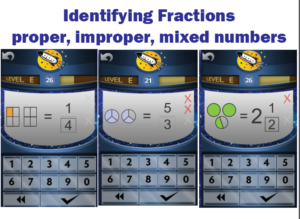When students initially learn about fractions they are often only shown proper fractions. As a result, they have a limited understanding of fractions and can be confused by improper fractions or mixed numbers. The Rocket Math programs (both Worksheet and Online) prevent this problem.  From the start, we teach students using examples of both proper and improper fractions as well as whole numbers and mixed numbers. Students learn to identify over 90 different fractions quickly and easily by getting lots of practice. Their understanding of fractions will deepen and become more flexible as they learn to recognize many examples of fractions.

### Learning Track 14: Equivalent Fractions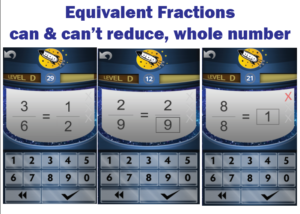Students will memorize the most common equivalent fractions with this Rocket Math Learning Track. They will also learn to identify a number of fractions, such as 2/9,  that do not “reduce” or for which there are no equivalent fractions in lower terms. Students also learn to recognize a fraction equal to 1 whole in its various forms. When students don’t instantly know the answer they are told the equivalent fraction and given practice on it. The computer gives help in the Online Game.  Their partner gives that help in the Worksheet Program. By the end of the program, students will learn over 90 equivalent fractions. This gives students an excellent start on being able to manipulate fractions quickly and easily.

### Learning Track 15: Factors & Primes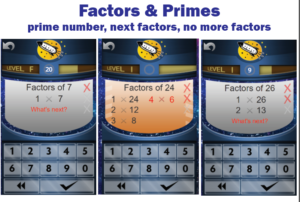Students are required to “find the factors” when dealing with unlike fractions and reducing fractions. Rocket Math Worksheet and Online Game teach students how to find factor pairs. Students learn how to find all the factor pairs and what they all are for many common numbers. They also learn to identify prime numbers and their characteristic of having only one and themselves as factors. Students learn the factor pairs in order and know the “last” factor pair when they see it. When the game asks “What’s next?” students can provide the next pair of factors or click the checkmark to indicate there are no more factors. When students go through this Learning Track they will no longer hesitate when asked for the factors of common numbers.

### Learning Track 16: Fraction & Decimal Equivalents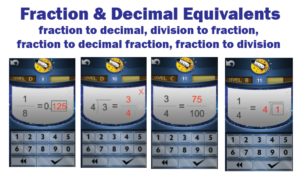Common fraction and decimal equivalents should not require a laborious process to “figure out.” Students should just know these, so this Learning Track in the Online Game allows them to memorize a bunch of common decimal and fraction equivalents. Having a facility with a lot of fraction and decimal equivalents means faster computation as well as a way to check their process when manipulating fractions and decimals. Students also learn another essential pre-algebra skill that often confuses them.  They learn to correctly and fluently translate a fraction into a division problem and vice-versa.

“Test Drive” any of the 16 Learning Tracks in our Online Game demo accounts.